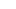# CAT Quant: On Graphs of Modulus Functions

(Photo: mingusmutter)

In this post, we are going to talk about two types of questions:

Find out the area of the bounded region

Find out the points with integer co-ordinates within the bounded region

Let us first look at some graphs.

|x| and -|x| These graphs will be mirror images of each other where the X-axis acts as the mirror|x+2| and |x-2| These will be like the graph of |x| shifted by two units to the left and right respectively|x| + 2 and |x| – 2 These will be like the graph of |x| shifted by two units to the upside and downside respectively|2x|and |x/2| These will be exactly the same as |x|, just the slope of the lines would be changed.If your graph is a modification of these ideas, then the question would not be so difficult.

Q1: Find out the area of the figure and integral points within the region formed by |2x+3|-5 and the X-axis

Here is what the graph would look like:As you can see, we have to find out the area of the triangle formed by the three black dots. Base of this triangle is 5 units and height of this triangle is also 5 units.

Area of this triangle would be=0.5*5*5=12.5

To find out the points that lie within the region, we need to consider the points which are inside the triangle.

If x=1, there is no such point

If x=0, there is (0,-1)

If x= -1, there are {(-1, -1),(-1,-2),(-1,-3)}

The figure is perfectly symmetric about x = -1.5. There are 4 points on the right side of it, so there will be 4 points on the left side of it. Total points=2*4 =8

Q2: Find out the area of the figure and integral points within the region formed by |2x|+|y|=6

In this example, we will have to consider both the positive and the negative values for both the modulus functions. The equations that we will get are

2x+y=6

2x–y=6

-2x+y=6

-2x–y=6 =>2x+y=-6

If we plot these on a graph, we will get the figureAs you can see, we have obtained a rhombus of side 3sqrt(5). Its diagonals are of length 12&6.

Area of the rhombus will be ½*12*6=36

To find points within the region, we need to consider points on X-axis and Y-axis

X axis=5{From -2 to +2}

Y axis=11{From -5 to +5}

Number of points on the axis =5+11–1=15 {The origin is common to the X and the Y Axis}

Number of points in quadrants =4*Points in one quadrant =4*4=16 {Points in first quadrant (1,1) ,(1,2), (1,3) ,(2,1)}

Total number of points=15+16=31

Ravi Handa, an alumnus of IIT Kharagpur, is the founder of www.handakafunda.com and has an online CAT coaching course.